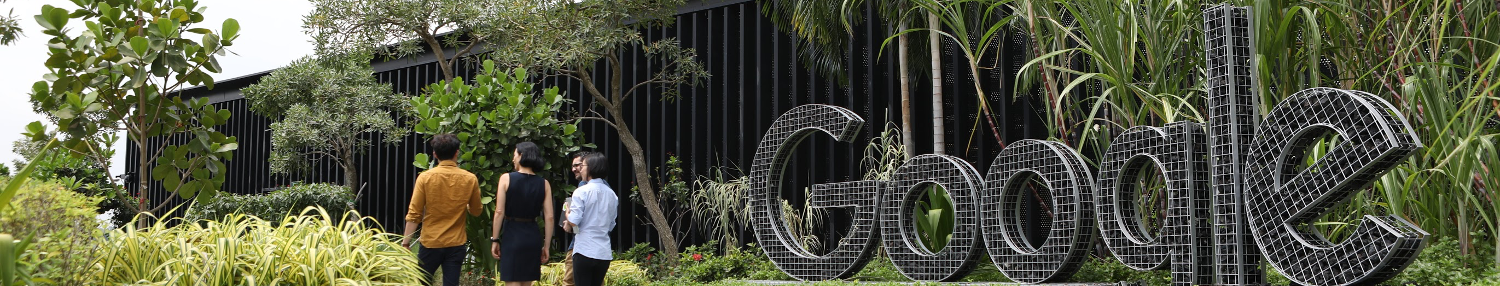## Pregunta de entrevista

Entrevista de Senior Software Engineer

-

# Write a probability formula to tell how many bits will be changed when 1 is added to a 32 bit binary number.

## Respuestas de entrevistas

11 respuestas

7

The probability of N bits being changed is (1/2)^N. The reason: the number of bits that will change depends on the position of the first zero that appears in the number. If the first zero is at the LSB, only one bit changes; if it is in the third position, the three bits upto the first zero change. Now, it boils down to the probability of finding the first zero. Assuming that the zeros and ones appear with equal probability in a given number, the probability of finding the first 0 in the Nth position is (1/2)^N. For more, look up the Geometric Random Variable.

Mythreya en

0

I think that you need to take into account that if you want to toggle 2 bits, you can only do if you flip bits from position 0..30. Toggling bit 31 is only going to toggle this bit no matter what. Therefore, you need to multiply (33-N)/32 to your proposed result, to keep this into account.

guillem en

1

@Mythreya's analysis is correct but incomplete. To get the expected value, you have to multiply the number of bits by their probability. Answer is Sigma{k/(2^k)} for k = 1 to 32.

Henry David Thoreau en

0

Using this approach, the answer is 2 bits will change on an average: https://answers.yahoo.com/question/index?qid=20110413165236AAU9tmO

Henry David Thoreau en

0

@Henry David Thoreau: The question is not asking for expected value, just the discrete density function. Also P(k=32) needs special handling.

Anónimo en

0

@Henry, no ... probability that at least 1 bit will change is (k/2^k); but, probability for all k bits to change, I guess, would still be 1/2^k. Right?

0

And, k=0 to 31 !!

.... en

0

k=1-32 p=k/2^(k-1)

... en

1

E(n) = 1 + E(n-1) / 2 where E(n) is the expected number of bit changes when 1 is added to an n-bit integer. E(1) = 1. E(2) = 1 + E(1) / 2 = 1.5. E(3) = 1 + E(2) / 2 = 1.75 We can verify it for n = 3. The possible values are as follows 000 -> 1 change 001 -> 2 change 010 -> 1 change 011-> 3 change 100-> 1 change 101-> 2 change 110-> 1 change 111-> 3 change Total changes: 14 Expected change = 14 / 8 = 1.75;

Mhret en

0

Anónimo en

0

If we just look at two bits - (b1,b2) f(b1) = { 1 b1=0; f(b2) if b1=1}, the probability of one bit changing is half. The probability of two bits changing is half * f(b2), which is half*half if b2=0 and half*half*f(b3) if b2=1. Therefore, for k=1-31 p(k) = 1/2^k and for p(k=32) = 1/2^k because when the 32nd bit flips there is nothing more to be done and the recursion stops

Anon en